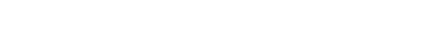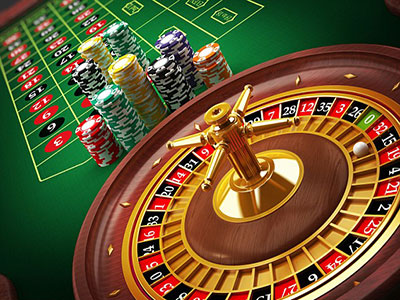#In online casino games the bets have a probability of winning. In online roulette these probabilities can be easily calculated using simple mathematics because all the outcomes are clearly known. In online blackjack the outcomes are so numerous that it requires computer simulation to estimate the probabilities. In online pokies all the outcomes are not known to the players, thus making it impossible for them to calculate the probabilities.

For a given probability of winning a bet, there is a mathematically correct payout that will lead to players just recovering their bet amounts in the long run. However, online casinos need to make money in order to meet their costs and provide nominal returns to their investors. Therefore the payout ratios for the various bets will be marginally less than the mathematically correct payouts. This difference is referred to as house edge. House edge can be defined as the percentage of a unit bet that on online casino expects to take in the long run. The calculation of the house edge for a simple roulette bet is explained.

One of the most common bets in online roulette is Red. This bet wins if the number called is red and loses if the number called is not red. The payout for this bet is 1 to 1. This means that for a bet of AU\$1 you will win AU\$1. The online casino will pay you AU\$2, which includes your AU\$1 bet amount. If the roulette wheel contained only the 18 red numbers and the 18 black numbers, the probability of winning in the Red bet would be 1/2. With a payout of 1 to 1 the odds would be mathematically correct and there would be no house edge.

But the European Roulette wheel has a 37th number, which is 0. The Red bet loses when 0 is called. Therefore the Red bet wins on 18 out of 37 numbers and has a probability of winning of 18/37. If you bet AU\$1 on Red you will win AU\$1 with a probability of 18/37 and will lose AU\$1 with a probability of 19/37. In the net, your expected loss on an AU\$1 bet will be AU\$(18/37 – 19/37), which works out to AU\$(1/37). When expressed as a percent of the bet amount this is 2.7%, which is the house edge for Red bet in European Roulette. Similar calculation for all other bets will reveal that all bets in European Roulette have a house edge of 2.7%.